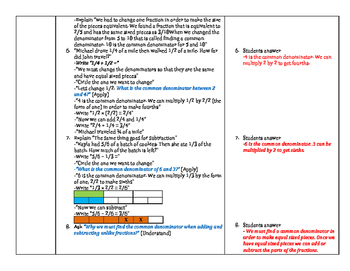# Adding/Subtracting Fractions & Mixed Numbers/Improper FractionsSubject
File Type

PDF

(737 KB|17 pages)
Product Rating
Standards
• Product Description
• StandardsNEW
This four day lesson sequence teaches the importance of finding common denominators, adding and subtracting fractions with unlike denominators, and converting mixed numbers and improper fractions. These lessons explain how to find common denominators, add/subtract fractions, and convert mixed numbers and improper fractions using pictures. These detailed lessons include standards, learning objectives, questions and answers, and differentiation.
Solve word problems involving addition and subtraction of fractions referring to the same whole, including cases of unlike denominators, e.g., by using visual fraction models or equations to represent the problem. Use benchmark fractions and number sense of fractions to estimate mentally and assess the reasonableness of answers. For example, recognize an incorrect result 2/5 + 1/2 = 3/7, by observing that 3/7 < 1/2.
Add and subtract fractions with unlike denominators (including mixed numbers) by replacing given fractions with equivalent fractions in such a way as to produce an equivalent sum or difference of fractions with like denominators. For example, 2/3 + 5/4 = 8/12 + 15/12 = 23/12. (In general, 𝘢/𝘣 + 𝘤/𝘥 = (𝘢𝘥 + 𝘣𝘤)/𝘣𝘥.)
Total Pages
17 pages
Included
Teaching Duration
4 days
Report this Resource to TpT
Reported resources will be reviewed by our team. Report this resource to let us know if this resource violates TpT’s content guidelines.